# GPA Formula – Excel & Google Sheets

This tutorial will demonstrate how to calculate GPA in Excel and Google Sheets.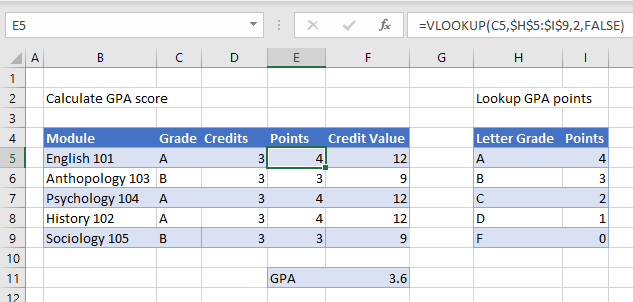To create a GPA calculator in Excel, we use several different Excel functions and calculations.

## VLOOKUP

First, we look up the number of points obtained in each module based on the grade received for the module.  We use the VLOOKUP Function to lookup the points from the lookup table.

``=VLOOKUP(C5,\$H\$5:\$I\$9,2,FALSE)``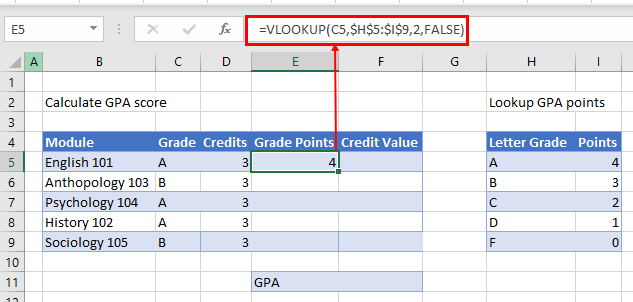Once we have the grade points for the first module, we can copy the formula down for the rest of the modules as we have used an absolute (\$) in the lookup range.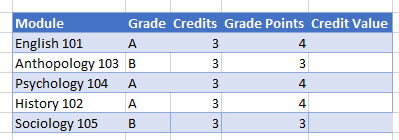Next, we work out the credit value for each of the modules.  We do this by multiplying the grade points received by the credit value.

``=D5*E5``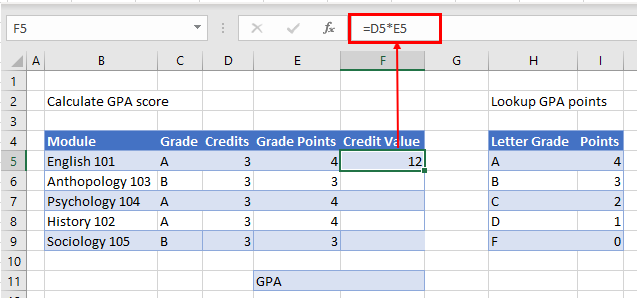We can then copy that formula down for the other modules to get the credit value received for each module.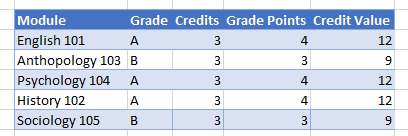Finally, we divide the sum of the credit value received by the sum of the credits to obtain the GPA value.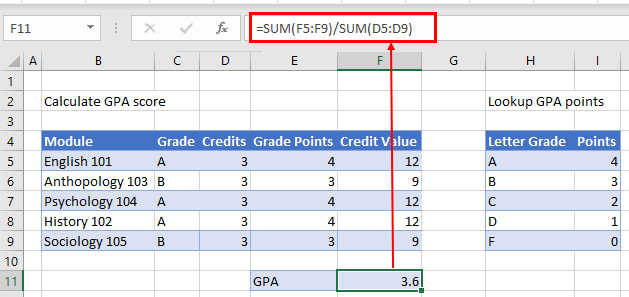## GPA Formula in Google Sheets

The examples shown above work the same way in Google Sheets as they do in Excel.

### VLOOKUP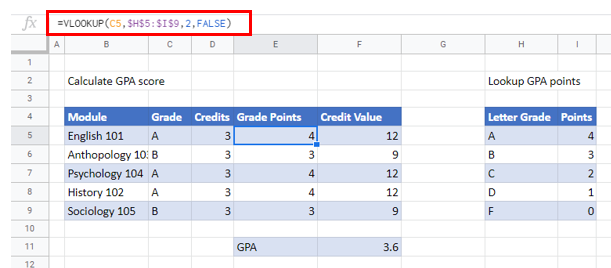### SUM to Calculate GPA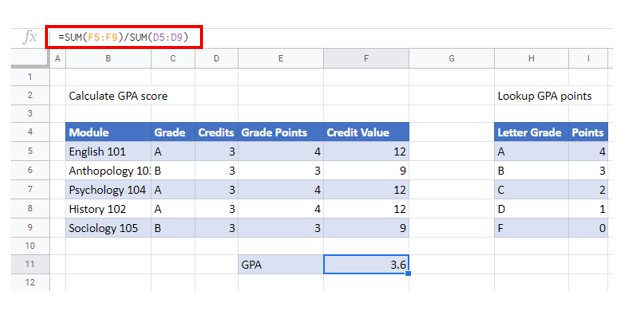### Excel Practice Worksheet

Practice Excel functions and formulas with our 100% free practice worksheets!